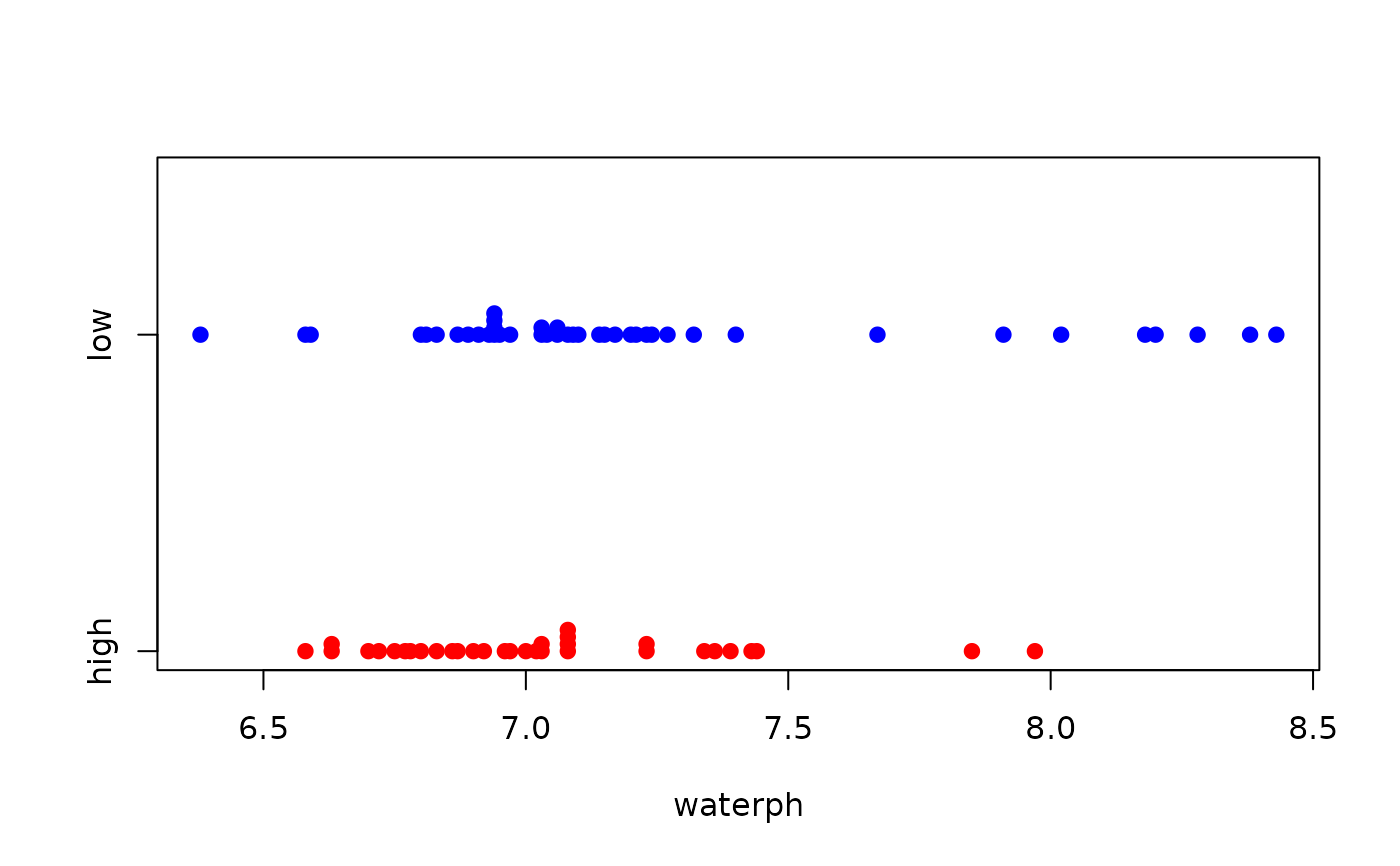Data for Exercises 6.40, 6.59, 7.10, and 7.35

Smokyph

## Format

A data frame/tibble with 75 observations on three variables

waterph

water sample pH level

code

charater variable with values low (elevation below 0.6 miles), and high (elevation above 0.6 miles)

elev

elevation in miles

## Source

Schmoyer, R. L. (1994), Permutation Tests for Correlation in Regression Errors, Journal of the American Statistical Association, 89, 1507-1516.

## Examples


summary(Smokyph$waterph) #> Min. 1st Qu. Median Mean 3rd Qu. Max. #> 6.380 6.880 7.030 7.140 7.235 8.430 tapply(Smokyph$waterph, Smokyph$code, mean) #> high low #> 7.039697 7.218095 stripchart(waterph ~ code, data = Smokyph, method = "stack", pch = 19, col = c("red", "blue"))t.test(Smokyph$waterph, mu = 7)
#>
#> 	One Sample t-test
#>
#> data:  Smokyph$waterph #> t = 2.7516, df = 74, p-value = 0.007452 #> alternative hypothesis: true mean is not equal to 7 #> 95 percent confidence interval: #> 7.038511 7.240689 #> sample estimates: #> mean of x #> 7.1396 #> SIGN.test(Smokyph$waterph, md = 7)
#>
#> 	One-sample Sign-Test
#>
#> data:  Smokyph\$waterph
#> s = 42, p-value = 0.2954
#> alternative hypothesis: true median is not equal to 7
#> 95 percent confidence interval:
#>  6.954832 7.085168
#> sample estimates:
#> median of x
#>        7.03
#>
#> Achieved and Interpolated Confidence Intervals:
#>
#>                   Conf.Level L.E.pt U.E.pt
#> Lower Achieved CI     0.9361 6.9600 7.0800
#> Interpolated CI       0.9500 6.9548 7.0852
#> Upper Achieved CI     0.9630 6.9500 7.0900
#>
t.test(waterph ~ code, data = Smokyph, alternative = "less")
#>
#> 	Welch Two Sample t-test
#>
#> data:  waterph by code
#> t = -1.859, df = 70.875, p-value = 0.03359
#> alternative hypothesis: true difference in means is less than 0
#> 95 percent confidence interval:
#>         -Inf -0.01845818
#> sample estimates:
#> mean in group high  mean in group low
#>           7.039697           7.218095
#>
t.test(waterph ~ code, data = Smokyph, conf.level = 0.90)
#>
#> 	Welch Two Sample t-test
#>
#> data:  waterph by code
#> t = -1.859, df = 70.875, p-value = 0.06718
#> alternative hypothesis: true difference in means is not equal to 0
#> 90 percent confidence interval:
#>  -0.33833836 -0.01845818
#> sample estimates:
#> mean in group high  mean in group low
#>           7.039697           7.218095
#>
if (FALSE) {
library(ggplot2)
ggplot2::ggplot(data = Smokyph, aes(x = waterph, fill = code)) +
geom_dotplot() +
facet_grid(code ~ .) +
guides(fill = FALSE)
}